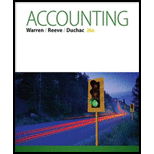Chapter 4, Problem 4.6BPE### Accounting (Text Only)

26th Edition
Carl Warren + 2 others
ISBN: 9781285743615

#### Solutions

Chapter
Section### Accounting (Text Only)

26th Edition
Carl Warren + 2 others
ISBN: 9781285743615
Textbook Problem
1 views

# Working capital and current ratioBalance sheet data for Brimstone Company follows: 2016 2015 Current assets $1,586,250$1,210,000 Current liabilities 705,000 550,000 a. Determine the working capital and current ratio for 2016 and 2015.b. Does the change in the current ratio from 2015 to 2016 indicate a favourable or an unfavorable trend?

(a)

To determine

Ratio analysis

It is the financial analysis tool for measuring the profitability, liquidity, capability and overall performance of a company.

Following are the two measures of liquidity:

1. 1. Current ratio: Current ratio is used to determine the relationship between current assets and current liabilities. The ideal current ratio is 2:1. The following formula is used to calculate current ratio.
2. 2. Working capital: Total current assets minus total current liabilities are the working capital of a company.

The working capital and current ratio for 2016 and 2015.

Explanation
 Details 2016 ($) 2015 ($) Current assets 1,586,250 1,210,000 Less: Current liabilities (705,000) (550,000) Working capital $881,250$660,000

Table (1)

Calculate the current ratio for 2015 and 2016.

Current ratio for 2015=Current assetsCurrentliabilities=$1,210,000$550,000=2

(b)

To determine

To Indicate: The change in current ratio form 2015 to 2016.

### Still sussing out bartleby?

Check out a sample textbook solution.

See a sample solution

#### The Solution to Your Study Problems

Bartleby provides explanations to thousands of textbook problems written by our experts, many with advanced degrees!

Get Started

#### Find more solutions based on key concepts